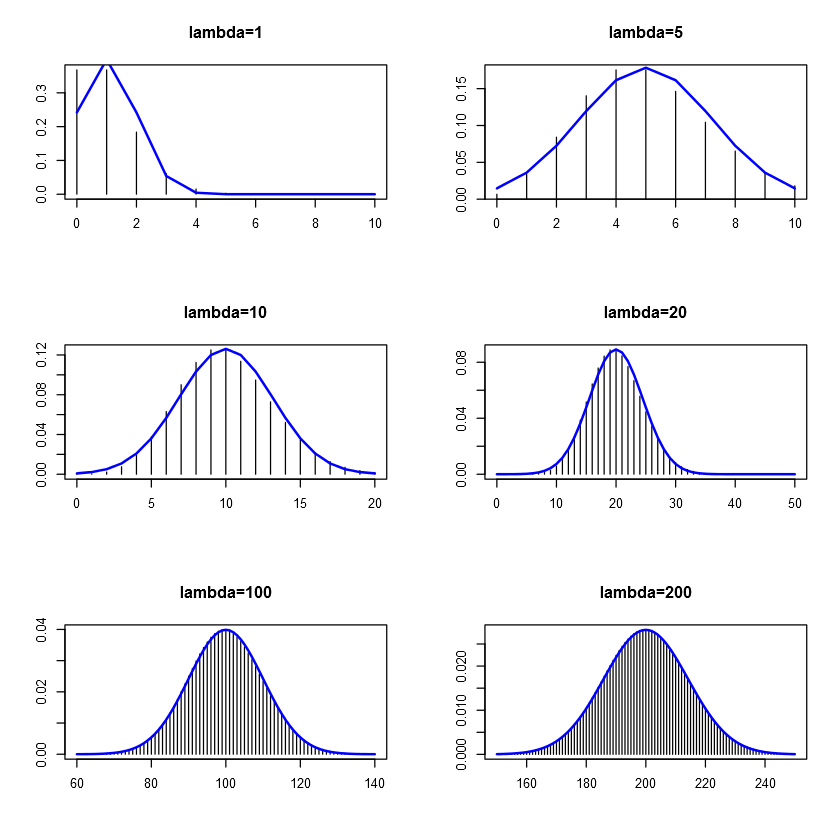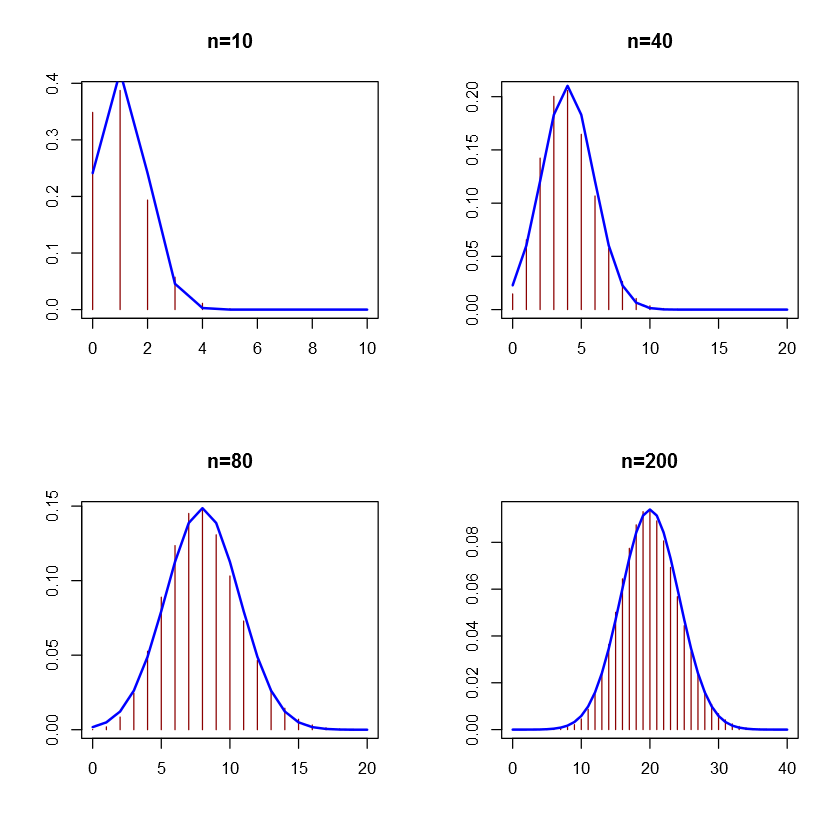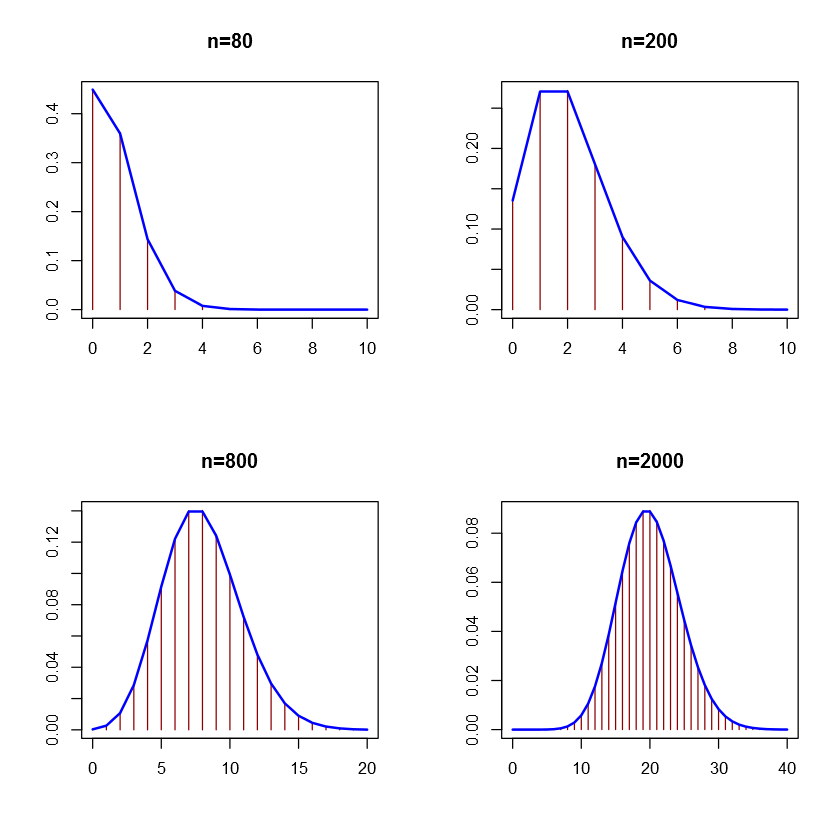# Fisseha Berhane, PhD

#### Data Scientist443-970-2353[email protected] CV Resume### Approximating poisson distribution with normal distribution¶

For sufficiently large values of λ, (say λ>1000), the normal distribution with mean λ and variance λ (standard deviation of lambda0.5 is an excellent approximation to the Poisson distribution. If λ is greater than about 10, then the normal distribution is a good approximation if an appropriate continuity correction is performed, i.e., P(X ≤ x), where (lower-case) x is a non-negative integer, is replaced by P(X ≤ x + 0.5).

In the figure below, we see that as λ increases, the poisson distibution becomes more and more like normal distribution.

The probability Pr(X=k) for k=0,1,2,...,n is calculated using R’s dpois(k,lambda) command.

Let's add a normal distribution with mean λ and variance λ.

In :
par(mfrow=c(3,2))

lambda<-c(1,5,10,20,100,200)

plot(0:10,dpois(0:10,lambda),type='h',xlab='',ylab='',main="lambda=1")
lines(0:10,dnorm(0:10,1,sqrt(1)),lwd=2,col="blue")

plot(0:10,dpois(0:10,lambda),type='h',xlab='',ylab='',main="lambda=5")
lines(0:10,dnorm(0:10,5,sqrt(5)),lwd=2,col="blue")

plot(0:20,dpois(0:20,lambda),type='h',xlab='',ylab='',main="lambda=10")
lines(0:20,dnorm(0:20,10,sqrt(10)),lwd=2,col="blue")

plot(0:50,dpois(0:50,lambda),type='h',xlab='',ylab='',main="lambda=20")
lines(0:50,dnorm(0:50,20,sqrt(20)),lwd=2,col="blue")

plot(60:140,dpois(60:140,lambda),type='h',xlab='',ylab='',main="lambda=100")
lines(60:140,dnorm(60:140,100,sqrt(100)),lwd=2,col="blue")

plot(150:250,dpois(150:250,lambda),type='h',xlab='',ylab='',main="lambda=200")
lines(150:250,dnorm(150:250,200,sqrt(200)),lwd=2,col="blue")### Approximating binomial distribution with normal distribution¶

A binomial distribution with at least 10 expected successes and 10 expected failures closely follows a normal distribution. If there are n trials with p probability of success and q probability of failure. This is clearly shown in the pattern of the figures below.

q=1-p

mean = np variance = npq

The number of success in n trials can be any between 0 and n. The probability Pr(X=k) for k=0,1,2,...,n is calculated using R’s dbinom(k,n,p) command.

Let's add a normal distribution with mean np and variance npq

In :
par(mfrow=c(2,2))

plot(0:10,dbinom(0:10,10,0.1),type="h",lwd=1,col="darkred",xlab="",ylab="",main="n=10")

lines(0:10,dnorm(0:10,(10*0.1),sqrt(10*0.1*0.9)),lwd=2,col="blue")

plot(0:20,dbinom(0:20,40,0.1),type="h",lwd=1,col="darkred",xlab="",ylab="",main="n=40")

lines(0:20,dnorm(0:20,(40*.1),sqrt(40*0.1*0.9)),lwd=2,col="blue")

plot(0:20,dbinom(0:20,80,0.1),type="h",lwd=1,col="darkred",xlab="",ylab="",main="n=80")

lines(0:20,dnorm(0:20,(80*.1),sqrt(80*0.1*0.9)),lwd=2,col="blue")

plot(0:40,dbinom(0:40,200,0.1),type="h",lwd=1,col="darkred",xlab="",ylab="",main="n=200")

lines(0:40,dnorm(0:40,(200*.1),sqrt(200*0.1*0.9)),lwd=2,col="blue")### Approximating binomial distribution with poisson distribution¶

When the probability of success p in a binomial distribution is very small and the number of trials n is very large, the binomial distribution can be approximated by posison distribution. Therefore, the Poisson distribution with parameter λ = np can be used as an approximation to B(n, p) of the binomial distribution if n is sufficiently large and p is sufficiently small. In the figures below, blue is poisson distribution generated using dpois function while orange is binomial distribution generated emloying dbinom function. As n becomes very large, both distributions become more like a normal distibution.

In :
par(mfrow=c(2,2))

plot(0:10,dbinom(0:10,80,0.01),type="h",lwd=1,col="darkred",xlab="",ylab="",main="n=80")
lines(0:10,dpois(0:10,80*0.01),lwd=2,col="blue")

plot(0:10,dbinom(0:10,200,0.01),type="h",lwd=1,col="darkred",xlab="",ylab="",main="n=200")

lines(0:10,dpois(0:10,200*0.01),lwd=2,col="blue")

plot(0:20,dbinom(0:20,800,0.01),type="h",lwd=1,col="darkred",xlab="",ylab="",main="n=800")

lines(0:20,dpois(0:20,800*0.01),lwd=2,col="blue")

plot(0:40,dbinom(0:40,2000,0.01),type="h",lwd=1,col="darkred",xlab="",ylab="",main="n=2000")

lines(0:40,dpois(0:40,2000*0.01),lwd=2,col="blue")